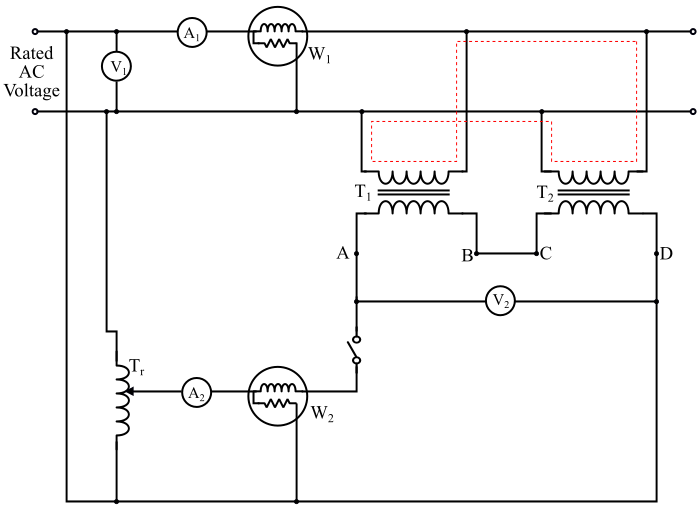# Back-to-Back Test (Sumpner’s Test) on Transformer

A full-load test on the large transformer is to be conducted to determine the maximum temperature rise of the transformer which is called as back-to-back test. The back-to-back test is also known as Sumpner’s test or regenerative test.

In case of small transformers, the full-load test is conveniently possible, but for large transformers, full load test is very difficult. It is because, a suitable load to consume full-load power of a large transformer may not be available. It will also be very expensive as a large amount of energy will be wasted in the load during the test.The figure shows the connection diagram of the back-to-back test. It requires two identical transformers T1 and T2. The primary windings of both the transformers are connected in parallel and being supplied at rated voltage and rated frequency. A voltmeter (V1), an ammeter (A1) and a wattmeter (W1) are connected to the input side. The secondary windings are connected in series with phase opposition polarities, which can be determined by the voltmeter V2. The range of the voltmeter V2 should be double of the rated secondary voltage of the either transformer.

In order to check whether the secondary windings are connected in series opposition or not, any two terminals (let B and C) are connected together and the voltage being measured between the remaining two terminals (here A and D) using the voltmeter V2. If the voltmeter V2 shows zero reading, then the two secondary windings are in series opposition and the terminals A and D can be used for the test. If the voltmeter V2 reads a value which is approximately equal to the double of the secondary voltage of either transformer, then secondary windings of both the transformers are acting in same direction. Then, the terminals A and C being connected together and the terminals B and D are to be used for the test.

Now, if the primary winding is connected to the supply, the total voltage across the two secondary windings which are connected in series would be zero. Thus, no current flows in secondary windings and hence they act as open circuited. Hence, the reading of the wattmeter (W1) gives the value of iron losses of both the transformers.

A low voltage is applied to the secondary circuit with the help of a regulating transformer Tr which is also excited by the main supply. The magnitude of this applied voltage is adjusted till the ammeter A2 reads full-load secondary current. This secondary current produces a full-load current in the primary windings which flows through the primary windings and the main busbar (shown by red coloured dotted lines). Therefore, the transformers act as operating on the fullload, hence the wattmeter W2 gives the value of full-load copper losses of both the transformers.

The total no-load current of the two transformers is measured by the ammeter A1. Therefore, in the back-to-back test, the two transformers are fully loaded, but the power drawn from the supply is that necessary to supply the losses of both the transformers.

The readings of the instruments connected in the circuit of the back-to-back test are as follows −

$$\mathrm{Ammeter\:𝐴_{1}\:reading = No\:load \:current\:of\:both\:transformers = 2𝐼_{0}}$$

$$\mathrm{Voltmeter\:𝑉_{1}\:reading = Applied \:rated\:primary\:voltage}$$

$$\mathrm{Wattmeter\:𝑊_{1}\:reading = Core \:losses\:of\:both\:transformers = 2𝑃_{i}}$$

$$\mathrm{Voltmeter\:𝑉_{2}\:reading = Total \:voltage\:across\:series\:connected\:secondaries}$$

$$\mathrm{Ammeter\:𝐴_{2}\:reading = full\:load\:secondary\:current\:of\:both\:transformers}$$

$$\mathrm{Wattmeter\:𝑊_{2}\:reading = Full \:load\:cu\:loss\:of\:both\:transformers = 2𝑃_{𝑐𝑢𝑓𝑙}}$$

Now, the temperature rise of both the transformers can be determined by operating the transformers back-to-back for a long time (say 36 to 48 hours) and measuring the temperature of the oil at periodic intervals of time (say every 1 hour).